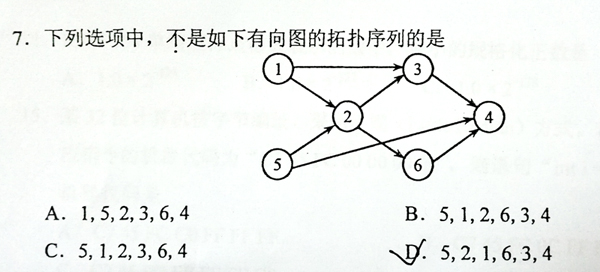# PAT 甲级 1146 Topological Order (25 分)(拓扑较简单，保存入度数和出度的节点即可)...

1146 Topological Order (25 分)

This is a problem given in the Graduate Entrance Exam in 2018: Which of the following is NOT a topological order obtained from the given directed graph? Now you are supposed to write a program to test each of the options.### Input Specification:

Each input file contains one test case. For each case, the first line gives two positive integers N (≤ 1,000), the number of vertices in the graph, and M (≤10,000), the number of directed edges. Then M lines follow, each gives the start and the end vertices of an edge. The vertices are numbered from 1 to N. After the graph, there is another positive integer K (≤ 100). Then K lines of query follow, each gives a permutation of all the vertices. All the numbers in a line are separated by a space.

### Output Specification:

Print in a line all the indices of queries which correspond to "NOT a topological order". The indices start from zero. All the numbers are separated by a space, and there must no extra space at the beginning or the end of the line. It is graranteed that there is at least one answer.

### Sample Input:

6 8
1 2
1 3
5 2
5 4
2 3
2 6
3 4
6 4
5
1 5 2 3 6 4
5 1 2 6 3 4
5 1 2 3 6 4
5 2 1 6 3 4
1 2 3 4 5 6


### Sample Output:

3 4

AC代码：

#include<bits/stdc++.h>
using namespace std;
int n,m,u,v;
int in,inx;
vector<int>out;
int main(){
cin>>n>>m;
memset(in,0,sizeof(in));
for(int i=1;i<=m;i++){
cin>>u>>v;
out[u].push_back(v);//保存出去的节点
in[v]++; //计算入度
}
int k;
cin>>k;
int a;
int num=0;
for(int i=0;i<k;i++){
int f=1;
memcpy(inx, in, sizeof(in));//将in拷贝给inx
for(int j=1;j<=n;j++){
cin>>u;
if(inx[u]!=0||f==0){
f=0;
continue;
}
for(int p=0;p<out[u].size();p++){//对受影响的节点的入度--
inx[out[u].at(p)]--;
}
}
if(!f){
a[++num]=i;
}
}
for(int i=1;i<=num;i++){
cout<<a[i];
if(i!=num) cout<<" ";
}
return 0;
} 

©️2019 CSDN 皮肤主题: 像素格子 设计师: CSDN官方博客